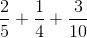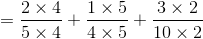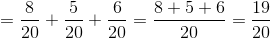# ISEE Middle Level Math : How to add fractions

## Example Questions

### Example Question #332 : Fractions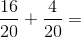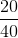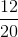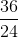Explanation: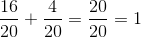### Example Question #333 : Fractions

Add: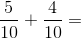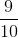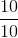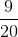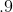Explanation:

Add the numerators and leave the denominators the same: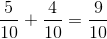Answer:### Example Question #334 : Fractions

Add: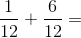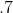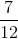Explanation:

Add the numerators and leave the denominators the same:Answer:### Example Question #335 : Fractions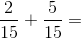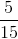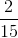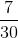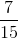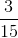Explanation:

Add the numerators and leave the denominators the same: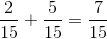Answer:### Example Question #336 : Fractions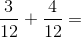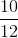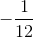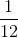Explanation:

Add the numerators and leave the denominators the same: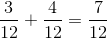Answer:### Example Question #337 : Fractions

Solve: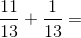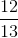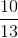Explanation:

Add the numerators and leave the denominators the same: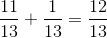Answer:### Example Question #338 : Fractions

Solve: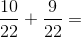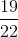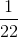Explanation:

Add the numerators and leave the denominators the same: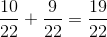Answer:### Example Question #339 : Fractions

Solve: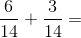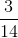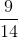Explanation:

Add the numerators and leave the denominators the same: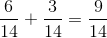Answer:### Example Question #340 : Fractions

Which of the following is the sum of sixty-seven tenths, sixty-seven hundredths, and sixty-seven ten-thousandths?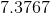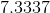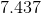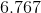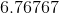Explanation:

Sixty-seven tenths, sixty-seven hundredths, and sixty-seven ten-thousandths can be rewritten as 6.7, 0.67, and 0.0067, respectively. Add, appending zeroes at the end of each number as needed: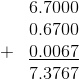### Example Question #41 : How To Add Fractions

Which of the following is the sum of two fifths, one fourth, and three tenths?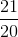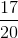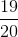Explanation:

We must express the three fractions as their equivalents with a common denominator. Since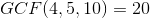, we rewrite each fraction in terms of twentieths, then add as follows: